## An animation has two scenes, each of them being one second long. In the first scene, an object moves along the path p⃗ 1(t)=(−8t2+3t,7t2+3,8

Question

An animation has two scenes, each of them being one second long. In the first scene, an object moves along the path p⃗ 1(t)=(−8t2+3t,7t2+3,8t2+6t−2) for 0≤t≤1. At the end of the scene, the object’s acceleration immediately becomes zero, and remains zero throughout the second scene. The vector-valued function p⃗ 2(t) for the object’s path in the second scene also uses 0≤t≤1, so that t=0 in the second scene is the same moment as t=1 in the first scene. What is p⃗ 2(t)?

in progress 0
2 months 2021-08-02T11:41:06+00:00 1 Answers 3 views 0

1. Answer: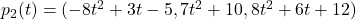Step-by-step explanation: Velocity is the first derivative of position function, so: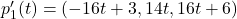Since in the second scene acceleration is zero, velocity must be constant, which means velocity in the second scene is the same as in the first scene, i.e.,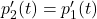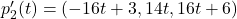To determine position function, integrate it: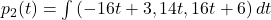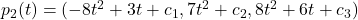Now, the “initial condition” is that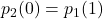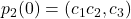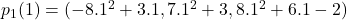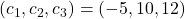Position function in the second scene is